# Hyperbolic metric

(diff) ← Older revision | Latest revision (diff) | Newer revision → (diff)

hyperbolic measure

A metric in a domain of the complex plane with at least three boundary points that is invariant under automorphisms of this domain.

The hyperbolic metric in the discis defined by the line element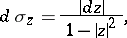where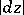is the line element of Euclidean length. The introduction of the hyperbolic metric inleads to a model of Lobachevskii geometry. In this model the role of straight lines is played by Euclidean circles orthogonal to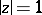and lying in; the circleplays the role of the improper point. Fractional-linear transformations ofonto itself serve as the motions in it. The hyperbolic length of a curvelying insideis defined by the formula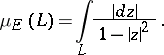The hyperbolic distance between two points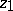andofis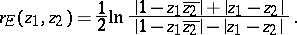The set of points ofwhose hyperbolic distance from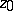,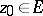, does not exceed a given number,, i.e. the hyperbolic disc inwith hyperbolic centre atand hyperbolic radius, is a Euclidean disc with centre other thanif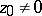.

The hyperbolic area of a domainlying inis defined by the formula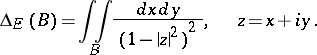The quantities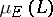,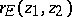and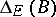are invariant with respect to fractional-linear transformations ofonto itself.

The hyperbolic metric in any domainof the-plane with at least three boundary points is defined as the pre-image of the hyperbolic metric inunder the conformal mappingofonto; its line element is defined by the formula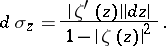A domain with at most two boundary points can no longer be conformally mapped onto a disc. The quantity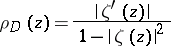is called the density of the hyperbolic metric of. The hyperbolic metric of a domaindoes not depend on the selection of the mapping function or of its branch, and is completely determined by. The hyperbolic length of a curvelocated inis found by the formula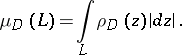The hyperbolic distance between two pointsandin a domainis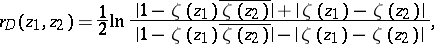where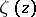is any function conformally mappingonto. A hyperbolic circle inis, as in the case of the disc, a set of points inwhose hyperbolic distance from a given point of(the hyperbolic centre) does not exceed a given positive number (the hyperbolic radius). If the domainis multiply connected, a hyperbolic circle inis usually a multiply-connected domain. The hyperbolic area of a domainlying inis found by the formulaThe quantities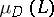,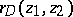and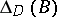are invariant under conformal mappings of(one of the main properties of the hyperbolic metric in).

How to Cite This Entry:
Hyperbolic metric. Encyclopedia of Mathematics. URL: http://encyclopediaofmath.org/index.php?title=Hyperbolic_metric&oldid=17817
This article was adapted from an original article by G.V. Kuz'mina (originator), which appeared in Encyclopedia of Mathematics - ISBN 1402006098. See original article